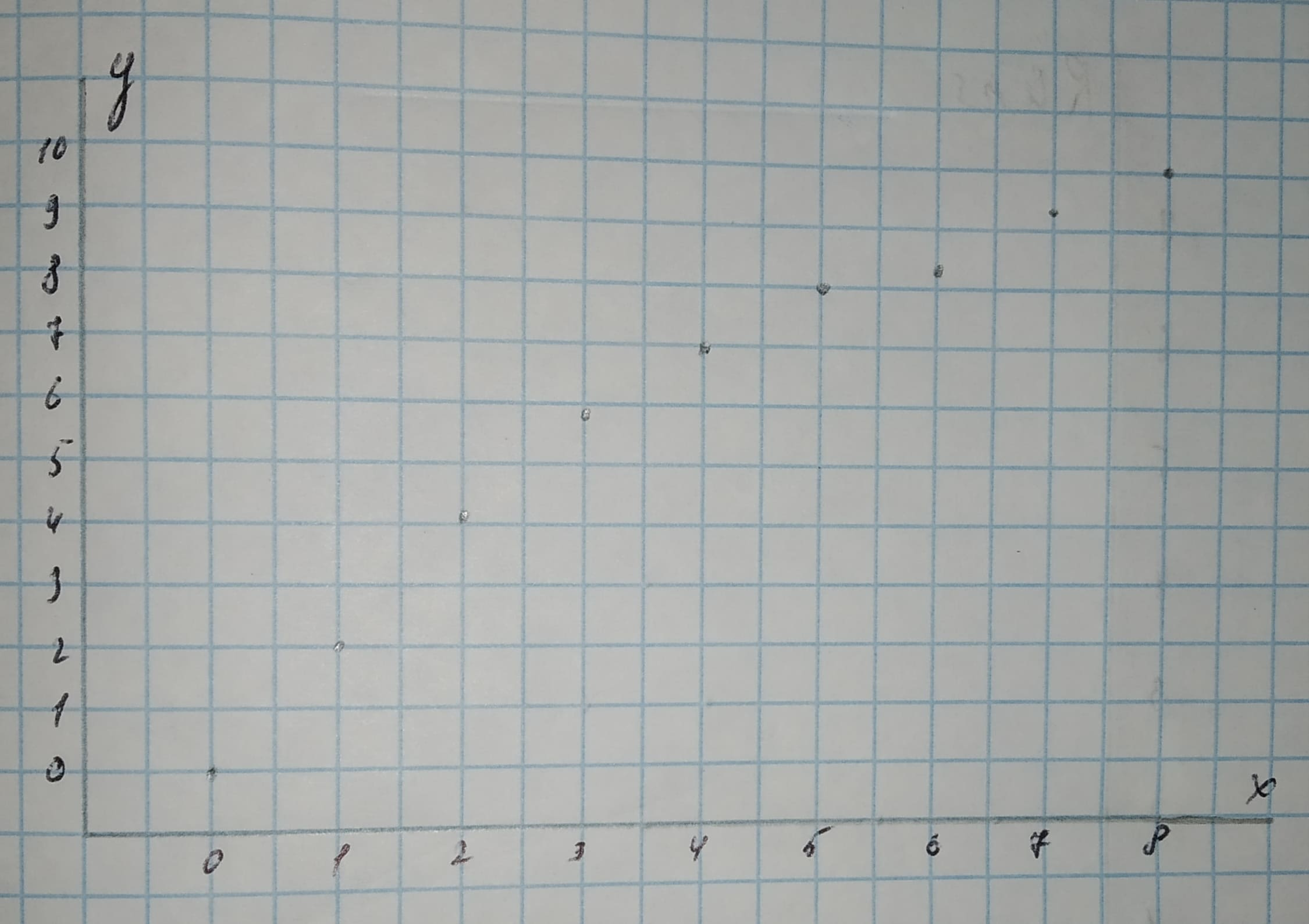pancha3

2021-02-19

Make a scatterplot for each set of data. Tell whether the data show a linear association or a nonlinear association.Caren

Step 1
Scatterplot
The first coordinate x in an ordered pair (x,y) is on the horizontal axis, while the second coordinate y is on the vertical axis.
The 2-values range from 0 to 8, thus an appropriate scale for the horizontal axis is from —1 to 9.
The y-values range from 0 to 10, thus an appropriate scale for the vertical axis is from —1 to 11.Step 2
We note that there is a slight curvature present in the scatterplot and thus the data show a nonlinear association.
Result:
Nonlinear assotiation.

Do you have a similar question?